Cyber Sale Extended 3 Days: ALL MCAT Products & Admissions Services

MCAT Content / Stoichiometry / Description Of Reactions By Chemical Equations

### Description of reactions by chemical equations

Topic: Stoichiometry

A chemical equation is the symbolic representation of a chemical reaction.

### Conventions for writing chemical equations

The reactants (the starting substances) are written on the left, and the products (the substances found in the chemical reaction) are written on the right. The coefficients next to the symbols of entities indicate the number of moles of a substance produced or used in the chemical reaction. Reactants and products are separated by an arrow symbol (“→” usually read aloud as “yields”). Each individual substance’s chemical formula is separated from others by a plus sign. The state of matter of each compound or molecule is indicated in subscript next to the compound by an abbreviation in parentheses. For example, a compound in the gas state would be indicated by (g), solid (s), liquid (l), and aqueous (aq). Aqueous means dissolved in water; it is a common state of matter for acids, bases, and dissolved ionic compounds.

### Balancing equations

Stoichiometry is the study of the relative quantities of reactants and products in chemical reactions and how to calculate those quantities. Chemical reactions are balanced by adding coefficients so that the number of atoms of each element is the same on both sides.

From a balanced equation, molar ratios can be derived. Molar rations state the proportions of reactants and products that are used and formed in a chemical reaction. Molar ratios can be derived from the coefficients of a balanced chemical equation.

As an example, the formula for the burning of methane can be written as follows:This equation indicates that oxygen and CH4 react to form H2O and CO2. It also indicates that two oxygen molecules are required for every methane molecule and that the reaction will form two water molecules and one carbon dioxide molecule for every methane and two oxygen molecules that react. The equation also identifies that all the compounds are in the gaseous state. The stoichiometric coefficients (the numbers in front of the chemical formulas) result from the law of conservation of mass and the law of conservation of charge.

Reactions can be classified in many ways, one way classifies reactions into 4 major groups based on the stoichiometry of the reactants and products. In a combination or synthesis reaction, separate molecules come together to form a new molecule (A+B -> AB). The opposite occurs in a decomposition reaction (AB -> A + B). In a single displacement (or single replacement) reaction, one element replaces another in a molecule (AB + C -> AC + B). Finally, in a. double displacement reaction, several reactant molecules exchange elements (AB + CD -> AC + BD).

### Balancing redox equations

Every balanced redox reaction is composed of two half-reactions: the oxidation half-reaction, and the reduction half-reaction. For example, look at the following redox reaction between iron and copper:

Fe + Cu2+ -> Fe2+ + Cu

In this reaction, iron is oxidized, and copper is reduced (or, iron is the reducing agent, and copper is the oxidizing agent.) We can split this reaction into two half-reactions. The oxidation half-reaction looks as follows: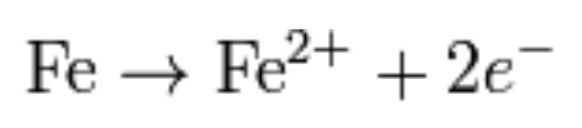This shows the oxidation of iron and the loss of two electrons. Notice that this equation is balanced in both mass and charge: we have one atom of iron on each side of the equation (mass is balanced), and the net charge on each side of the equation is equal to zero (charge is balanced).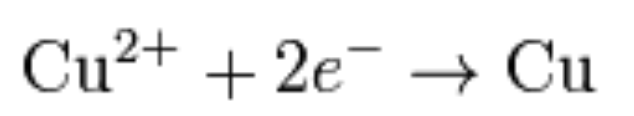This half-reaction explicitly shows the copper (II) ion gaining two electrons. Note again that the equation is balanced in mass and charge. Now that we have our two balanced half-reactions, we can combine them to get the full redox reaction: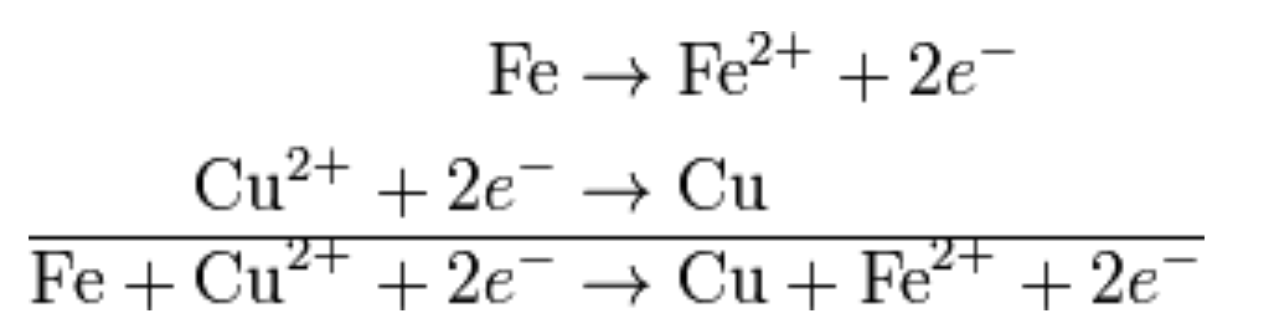Note that the two electrons on each side of the equation cancel out. This is very important because the final balanced equation for any redox reaction should never contain any electrons.

Many redox reactions occur in aqueous solution—in water. Because of this, in many cases, H2O or a fragment of an H2O molecule (H+ or OH, in particular) can participate in the redox reaction. As such, we need to learn how to incorporate the solvent into a balanced redox equation.

Consider the following oxidation half-reaction in aqueous solution, which has one Cr atom on each side: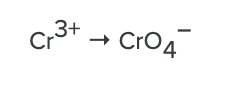Here, the Cr atom is going from the +3 to the +7 oxidation state. To do this, the Cr atom must lose four electrons. Let us start by listing the four electrons as products: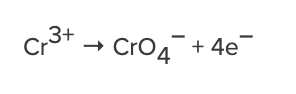But where do the O atoms come from? They come from water molecules or a common fragment of a water molecule that contains an O atom: the OH ion. When we balance this half-reaction, we should feel free to include either of these species in the reaction to balance the elements. Let us use H2O to balance the O atoms; we need to include four water molecules to balance the four O atoms in the products:This balances the O atoms but now introduces hydrogen to the reaction. We can balance the H atoms by adding an H+ ion, which is another fragment of the water molecule. We need to add eight H+ ions to the product side: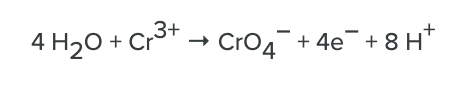The Cr atoms are balanced, the O atoms are balanced, and the H atoms are balanced; if we check the total charge on both sides of the chemical equation, they are the same (3+, in this case). This half-reaction is now balanced, using water molecules and parts of water molecules as reactants and products.

If a reaction occurs in an acidic environment, you can balance the redox equation as follows:

1. Write the oxidation and reduction half-reactions, including the whole compound involved in the reaction—not just the element that is being reduced or oxidized.
2. Balance both reactions for all elements except oxygen and hydrogen.
3. If the oxygen atoms are not balanced in either reaction, add water molecules to the side missing the oxygen.
4. If the hydrogen atoms are not balanced, add hydrogen ions (H+) until the hydrogen atoms are balanced.
5. Multiply the half-reactions by the appropriate numbers so that they both have equal numbers of electrons.
6. Add the two equations to cancel out the electrons to balance the equation.

### Limiting Reagents

The reagent that limits how much product is produced (the reactant that runs out first) is known as the limiting reagent. Given the balanced chemical equation that describes the reaction, there are several ways to identify the limiting reagent.

One way to determine the limiting reagent is to compare the mole ratio of the amount of reactants used. This method is most useful when there are only two reactants. One reactant (A) is chosen, and the balanced chemical equation is used to determine the amount of the other reactant (B) necessary to react with A. If the amount of B actually present exceeds the amount required, then B is in excess, and A is the limiting reagent. If the amount of B present is less than is required, then B is the limiting reagent.

To begin, the chemical equation must first be balanced. The law of conservation states that the quantity of each element does not change over the course of a chemical reaction. Therefore, the chemical equation is balanced when the amount of each element is the same on both the left and right sides of the equation. Next, convert all given information (typically masses) into moles, and compare the mole ratios of the given information to those in the chemical equation.

For example: What would be the limiting reagent if 75 grams of C2H3Br3 reacted with 50.0 grams of O2 in the following reaction:First, convert the values to moles:It is then possible to calculate how much C2H3Br3 would be required if all the O2 is used up:This demonstrates that 0.567 mol C2H3Br3 is required to react with all the oxygen. Since there is only 0.28 mol C2H3Br3 present, C2H3Br3 is the limiting reagent.

Another method of determining the limiting reagent involves the comparison of product amounts that can be formed from each reactant. This method can be extended to any number of reactants more easily than the previous method. Again, begin by balancing the chemical equation and by converting all the given information into moles. Then use stoichiometry to calculate the mass of the product that could be produced for each individual reactant. The reactant that produces the least amount of product is the limiting reagent.

### Yield

The per cent yield of a reaction measures the reaction’s efficiency. It is the ratio between the actual yield and the theoretical yield. The theoretical yield for a reaction is calculated based on the limiting reagent. This allows researchers to determine how much product can actually be formed based on the reagents present at the beginning of the reaction. The actual yield will never be 100 per cent due to limitations.For example, consider the preparation of nitrobenzene (C6H5NO2), starting with 15.6g of benzene (C6H6) in excess of nitric acid (HNO3):In theory, therefore, if all C6H6 were converted to product and isolated, 24.6 grams of product would be obtained (100 per cent yield). If 18.0 grams were actually produced, the per cent yield could be calculated:Practice Questions

Qualitative analysis of contaminated water supply

MCAT Official Prep (AAMC)

Chemistry Question Pack Passage 4 Question 23

Chemistry Question Pack Question 54

Chemistry Question Pack Passage 11 Question 60

Chemistry Question Pack Passage 16 Question 86

Practice Exam 1 C/P Section Passage 9 Question 48

Practice Exam 1 C/P Section Passage 9 Question 49

Practice Exam 1 C/P Section Passage 10 Question 54

Practice Exam 3 C/P Section Passage 3 Question 16

Practice Exam 3 C/P Section Passage 5 Question 21

Practice Exam 3 C/P Section Passage 6 Question 32

Key Points

• In a chemical equation, the reactants are written on the left, and the products are written on the right.

• The coefficients next to the symbols of entities indicate the number of moles of a substance produced or used in the chemical reaction.

• The reactants and products are separated by an arrow, usually read aloud as “yields.”

• Chemical equations should contain information about the state properties of products and reactants, whether aqueous (dissolved in water — aq), solid (s), liquid (l), or gas (g).

• One possible classification system classifies reactions as combination, decomposition, single displacement or double displacement.

• Reducing agents get oxidized, and therefore lose electrons.

• Oxidizing agents get reduced and therefore gain electrons.

• Remember the mnemonic device OIL RIG—”Oxidation Involves Loss” and “Reduction Involves Gain” to distinguish between oxidizing and reducing agents.

• The limiting reagent is the reactant that is used up completely. This stops the reaction, and no further products are made. One way to determine the limiting reagent is to compare the mole ratios of the amounts of reactants used. This method is most useful when there are only two reactants.

• The theoretical yield for a reaction is calculated based on the limiting reagent. This allows researchers to determine how much product can actually be formed based on the reagents present at the beginning of the reaction.

Key Terms

Stoichiometry: the field of chemistry that is concerned with the relative quantities of reactants and products in chemical reactions and how to calculate those quantities

Ratio: the ratio of the coefficients of the products and reactants in a balanced reaction. This ratio can be used to calculate the amount of products or reactants produced or used in a reaction

Combination reaction: A + B -> AB

Decomposition reaction: AB -> A + B

Single displacement reaction: AB + C -> AC + B

Double displacement reaction: AB + CD -> AC + BD

Reduction: a reaction in which electrons are gained, and oxidation state is reduced, often by the removal of oxygen or the addition of hydrogen

Half-reactions: one of the two constituent parts of any redox reaction in which only oxidation or reduction is shown

Oxidation: a reaction in which an element’s atoms lose electrons and its oxidation state increases

Limiting reagent: the reactant in a chemical reaction that is consumed first; prevents any further reaction from occurring

Theoretical yield: the amount of product that could possibly be produced in a given reaction, calculated according to the starting amount of the limiting reagent

Actual yield: the amount of product actually obtained in a chemical reaction

Chemical equation: a symbolic representation of a chemical reaction; reactants are represented on the left and products on the right

Reactant: the starting materials in a chemical reaction

Product: the compounds produced by a chemical reaction

Law of conservation of mass: matter cannot be created or destroyed. Therefore, in a closed system, the mass of the reactants must equal the mass of the products

Coefficient: a constant by which an algebraic term is multiplied

Billing Information